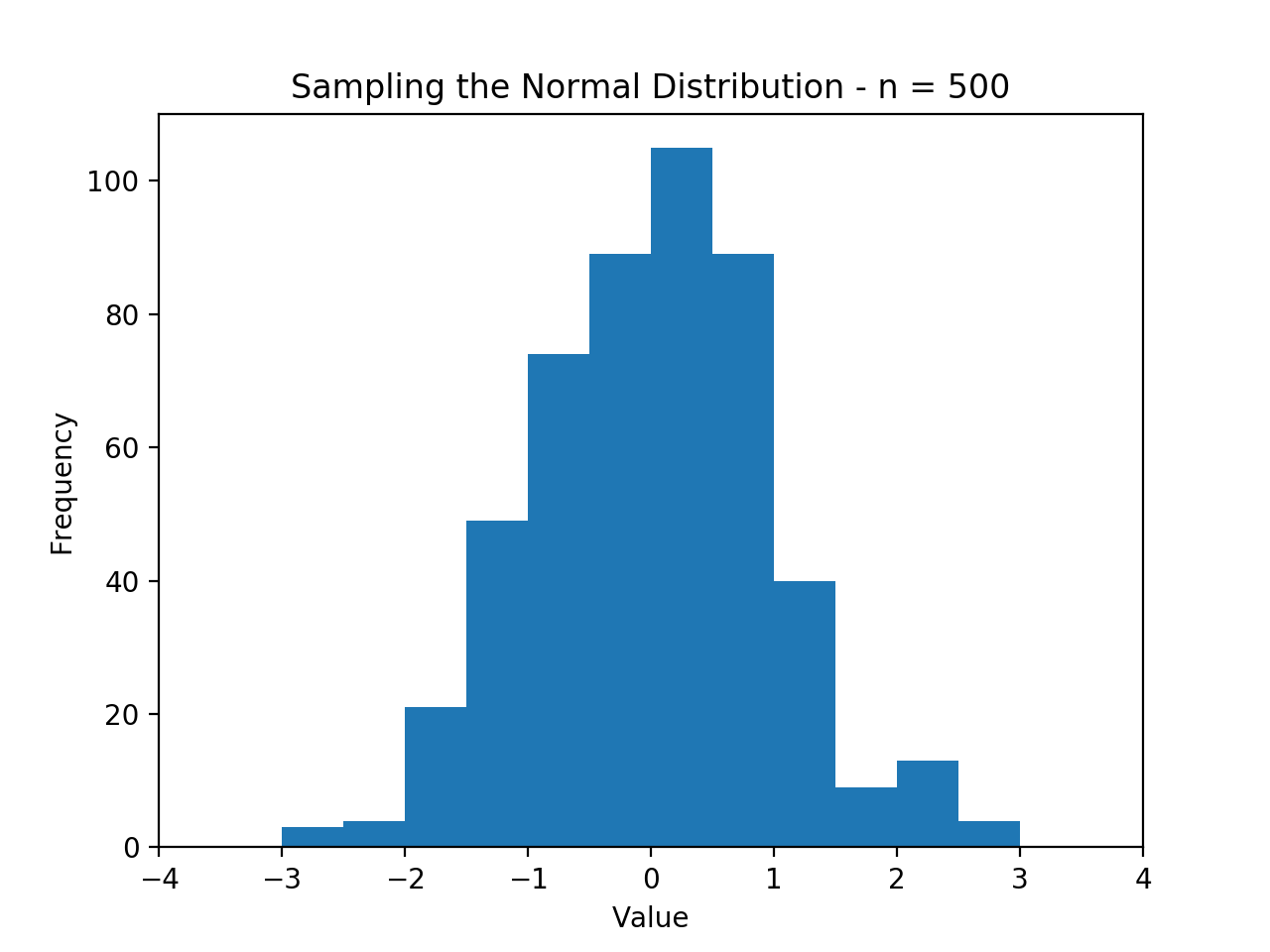# Animations

Matplotlib supports animations in addition to static images. Recall that the “backend” is what renders each plot to the screen, and animations heavily reply on support from the backend. The backend provided by the %matplotlib notebook magic function is one that offers support for animations.

The demonstration below shows how a histogram is constructed by repeatedly samping from a normal distribution and adding the next sample to the figure.

%matplotlib notebook

import matplotlib.pyplot as plt
import numpy as np

n = 500
x = np.random.randn(n)
print(x[:5])

[-0.16869264  0.25741228 -1.83127465  0.6417844  -0.41111246]


Setting up animations in matplotlib requires a few items to be set up. The first important object is called FuncAnimation, which builds an animation by iteratively calling a function defined below, update.

The function defined below performs a few steps.

• If the current frame (given by curr) is the last frame, it stops the animation.
• Otherwise, it:
• Clears the axis.
• Plots the first curr items in x in the histogram.
• Then, completes the rest of the histogram setup.
import matplotlib.animation as animation

def update(curr):
if curr == n:
a.event_source.stop()

plt.cla()
bins = np.arange(-4, 4, 0.5)

plt.hist(x[:curr],
bins=bins)

plt.axis([-4,4,0,110])
title = 'Sampling the Normal Distribution - n = {}'.format(curr)
plt.gca().set_title(title)
plt.gca().set_ylabel('Frequency')
plt.gca().set_xlabel('Value')


Next, create a figure in the normal way, and pass it to animation.FuncAnimation. The other parameters to this function are the update function we just defined, and an interval between updates, set below to 100 milliseconds.

The image shown on my website, below, is static but in a jupyter notebook is animated.

fig = plt.figure()
a = animation.FuncAnimation(fig,
update,
interval=100)

<IPython.core.display.Javascript object>It is also possible to save clips and render the clips inline, even in HTML, using the %matplotlib inline backend. This will be demonstrated next.